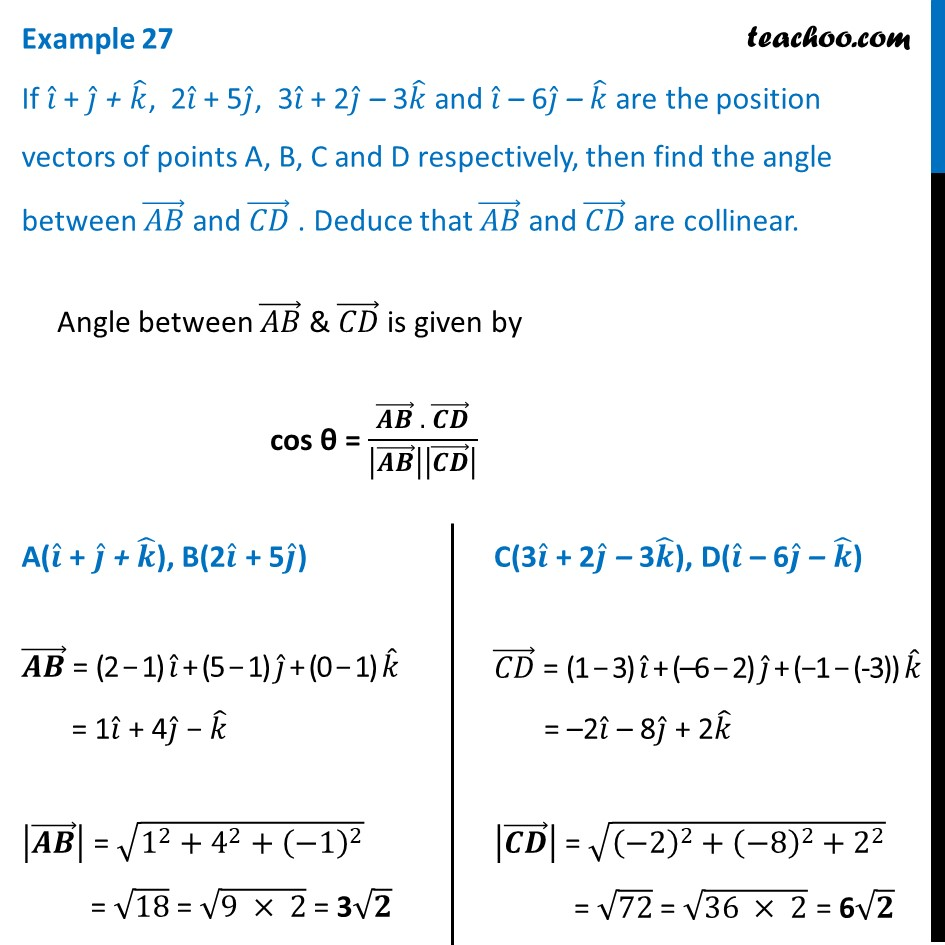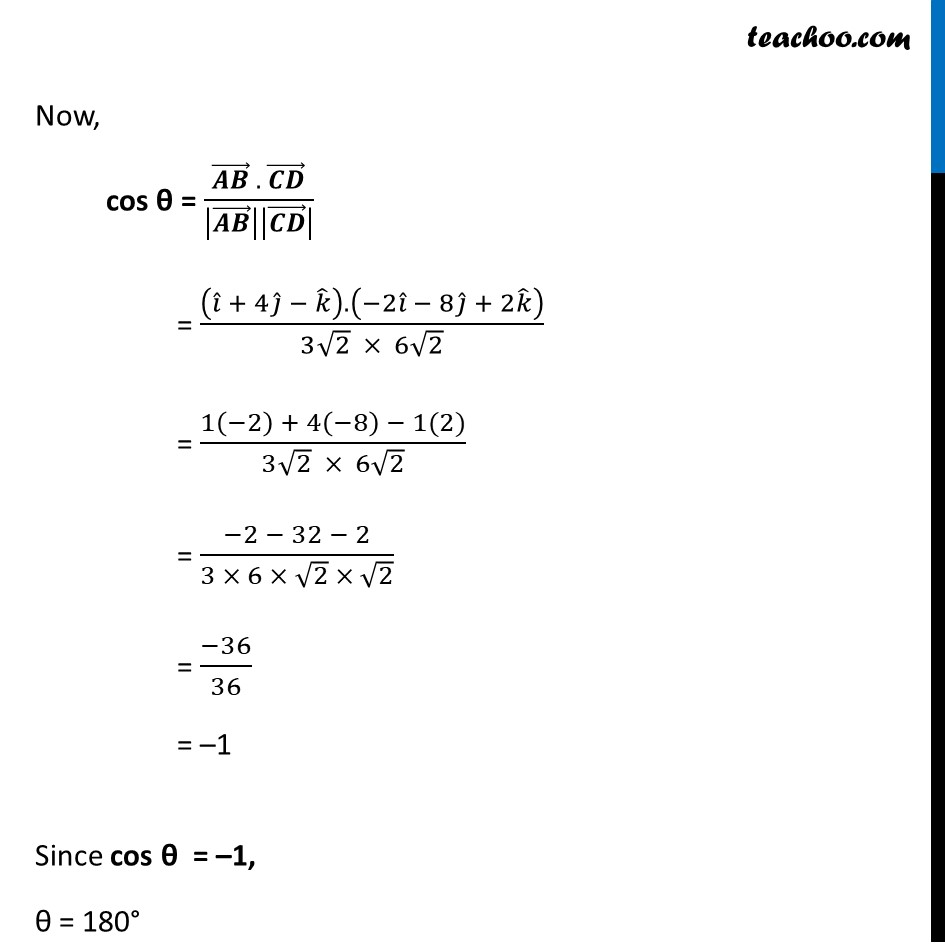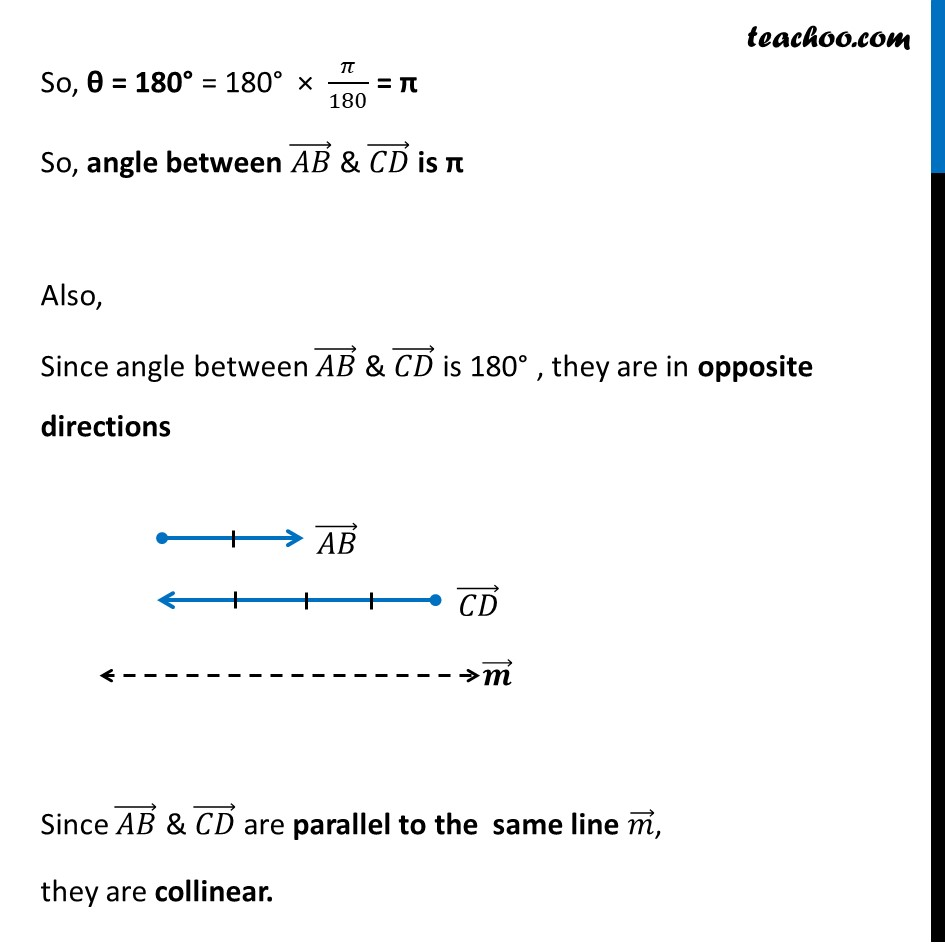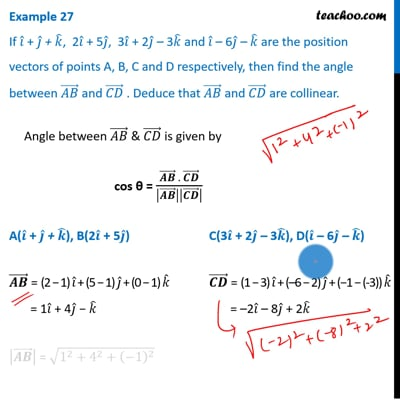Examples

Chapter 10 Class 12 Vector Algebra
Serial order wiseThis video is only available for Teachoo black users

Introducing your new favourite teacher - Teachoo Black, at only ₹83 per month

### Transcript

Example 27 If 𝑖 ̂ + 𝑗 ̂ + 𝑘 ̂, 2𝑖 ̂ + 5𝑗 ̂, 3𝑖 ̂ + 2𝑗 ̂ – 3𝑘 ̂ and 𝑖 ̂ – 6𝑗 ̂ – 𝑘 ̂ are the position vectors of points A, B, C and D respectively, then find the angle between (𝐴𝐵) ⃗ and (𝐶𝐷) ⃗ . Deduce that (𝐴𝐵) ⃗ and (𝐶𝐷) ⃗ are collinear.Angle between (𝐴𝐵) ⃗ & (𝐶𝐷) ⃗ is given by cos θ = ((𝑨𝑩) ⃗ . (𝑪𝑫) ⃗)/|(𝑨𝑩) ⃗ ||(𝑪𝑫) ⃗ | A(𝒊 ̂ + 𝒋 ̂ + 𝒌 ̂), B(2𝒊 ̂ + 5𝒋 ̂) (𝑨𝑩) ⃗ = (2 − 1) 𝑖 ̂ + (5 − 1) 𝑗 ̂ + (0 − 1) 𝑘 ̂ = 1𝑖 ̂ + 4𝑗 ̂ − 𝑘 ̂ |(𝑨𝑩) ⃗ | = √(1^2+4^2+(−1)^2 ) = √18 = √(9 × 2) = 3√𝟐 C(3𝒊 ̂ + 2𝒋 ̂ – 3𝒌 ̂), D(𝒊 ̂ – 6𝒋 ̂ – 𝒌 ̂) (𝐶𝐷) ⃗ = (1 − 3) 𝑖 ̂ + (–6 − 2) 𝑗 ̂ + (−1 − (-3)) 𝑘 ̂ = –2𝑖 ̂ – 8𝑗 ̂ + 2𝑘 ̂ |(𝑪𝑫) ⃗ | = √((−2)^2+(−8)^2+2^2 ) = √72 = √(36 × 2) = 6√𝟐 Now, cos θ = ((𝑨𝑩) ⃗ . (𝑪𝑫) ⃗)/|(𝑨𝑩) ⃗ ||(𝑪𝑫) ⃗ | = ((𝑖 ̂ + 4𝑗 ̂ − 𝑘 ̂ ).(−2𝑖 ̂ − 8𝑗 ̂ + 2𝑘 ̂ ))/(3√2 × 6√2) = (1(−2) + 4(−8) − 1(2))/(3√2 × 6√2) = (−2 − 32 − 2)/(3 × 6 × √2 × √2) = (−36)/36 = –1 Since cos θ = –1, θ = 180° So, θ = 180° = 180° × 𝜋/180 = π So, angle between (𝐴𝐵) ⃗ & (𝐶𝐷) ⃗ is π Also, Since angle between (𝐴𝐵) ⃗ & (𝐶𝐷) ⃗ is 180° , they are in opposite directions Since (𝐴𝐵) ⃗ & (𝐶𝐷) ⃗ are parallel to the same line 𝑚 ⃗, they are collinear.